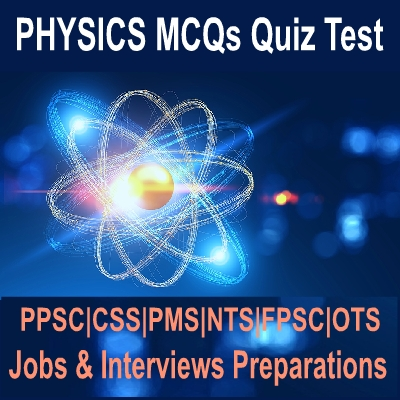Sunday, 12 January 2020

# PPSC Physics Subject Specialist Past Papers MCQs

If you have applied for Teaching jobs test in PPSC, FPSC, CSS, PMS, OTS, CTS, NTS and others exams specialist for teaching then these MCQs with help you for Preparations of exams tests of Physics. All of these Past Papers important repeated MCQs are helpful for Subject Specialist of Physics Teacher jobs.Physics MCQs for entry test preparations

Physics MCQs Online Test Quiz Preparations are important for MCAT, ECAT, GAT and others comparative exams in which Physics Question Answers are included for the tests.

### Physics Online Solved Question Answers MCQs For Teaching Jobs Test

Q.1: The operation of a transistor requires--------- ?

1. That the emitter be heated
2. That the base be heated
3. That the collector be heated
4. None of the above

D

Q.2: The unit of coefficient of linear expansion or volume expansion is--------

1. K
2. K-1
3. K-2
4. None

B

Q.3: The rectangular voltage is an example of---------- ?

1. An analogue waveform
2. Continuous wave form
3. Electronic waveform
4. A digital waveform

D

Q.4: The pressure exerted by a column of mercury 76cm high and at 0°C is called-------- ?

1. 1 atmosphere
2. 1 Newton per square meter
3. 1 Pascal
4. data is insufficient

A

Q.5: Which one is not an example of adiabatic process ?

1. rapid escape of air from a burst tyre
2. rapid expansion of air
3. conversion of water into ice in refrigerator
4. cloud formation in the atmosphere

C

Q.6: Which one is true for internal energy ?

1. It is sum of all forms of energies associated with molecules of a system
2. It is a state function of a system
3. It is proportional to transnational K.E of the molecules
4. All are correct

D

Q.7: Average translational kinetic energy per molecule of an ideal gas is given by-------- ?

1. 3NaT/2R
2. 2NaT/3
3. 3RT/2Na
4. 3Na/2RT

C

Q.8: The performance of a refrigerator is described by--------- ?

1. efficiency
2. coefficient of performance
3. both A and B
4. not described

B

Q.9: Two identical coaxial circular loops carry equal currents in the same direction. If the loops approach each other the current in------- ?

1. Each increase
2. Each decrease
3. Each remains the same
4. One increases whereas that in the other decreases

B

Q.10: Cosθi^ + Sinθj^ is a---------- ?

1. Vector
2. Position Vector
3. Vector in the direction at angle θ with an x-axis
4. The unit vector in the direction at angle θ with an x-axis

D

Q.11: Which of the following pairs does not have identical dimensions ?

1. Torque and energy
2. Momentum and impulse
3. Energy and work
4. Mass and moment of inertia

D

Q.12: The magnitude of i^. (j^ x k^) is---------- ?

1. 0
2. 1
3. -1
4. i^

B

Q.13: The magnitude of the cross product is equal to the dot product between them. The angle between the two vectors is--------- ?

1. 30°
2. 45°
3. 60°
4. 180°

B

Q.14: If A=B, which of the following is not correct ?

1. A.B = A^ B^
2. |A| = |B|
3. |A^| = |B^|
4. AB^ = BA^

A

Q.15: In the nucleus of uranium, the number of neutrons will be -------- ?

1. 92
2. 235
3. 143
4. Different for different isotopes

D

Q.16: Which one of the following radiation possesses maximum penetrating power ?

1. X-rays
2. B-rays
3. γ-rays
4. All have the equal penetrating powe

C

Q.17: The charge on an electron was determine by --------- ?

1. Ampere
2. Maxwell
3. Millikan
4. Thomson

C

Q.18: Nuclear forces exist between --------- ?

1. Proton-proton
2. Proton-neutron
3. Neutron-neutron
4. All of the above

D

Q.19: The number of significant figures in 5.400 is-------- ?

1. 3
2. 5
3. 2
4. None

D

Q.20: Geostationary satellite completes one rotation around the earth in---------- ?

1. 3 hours
2. 6 hours
3. 12 hours
4. 24 hours

D

Share: